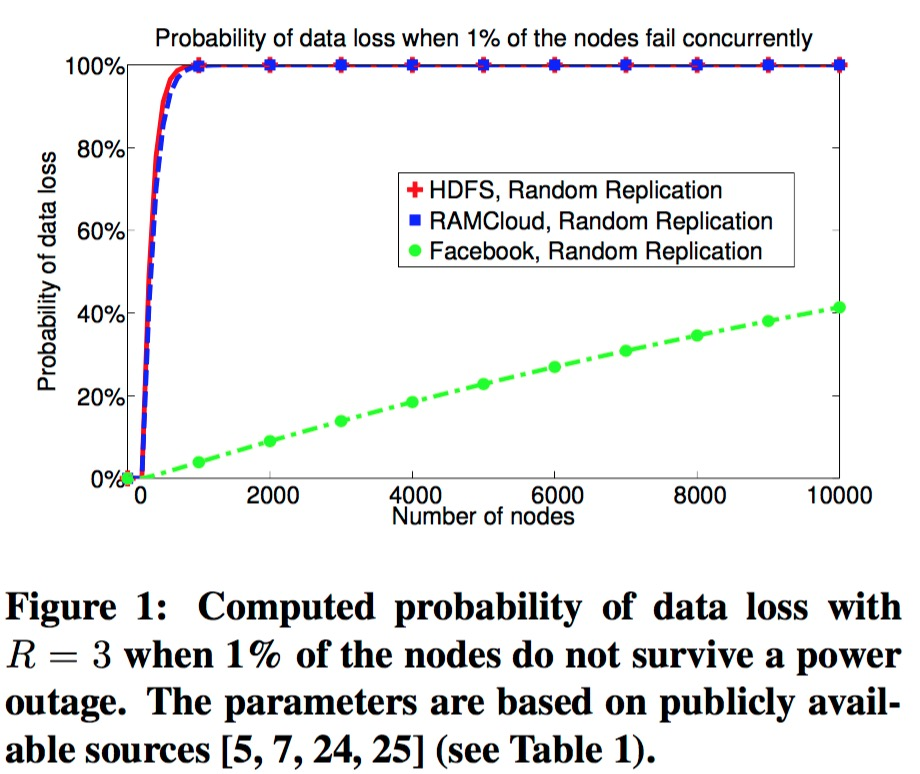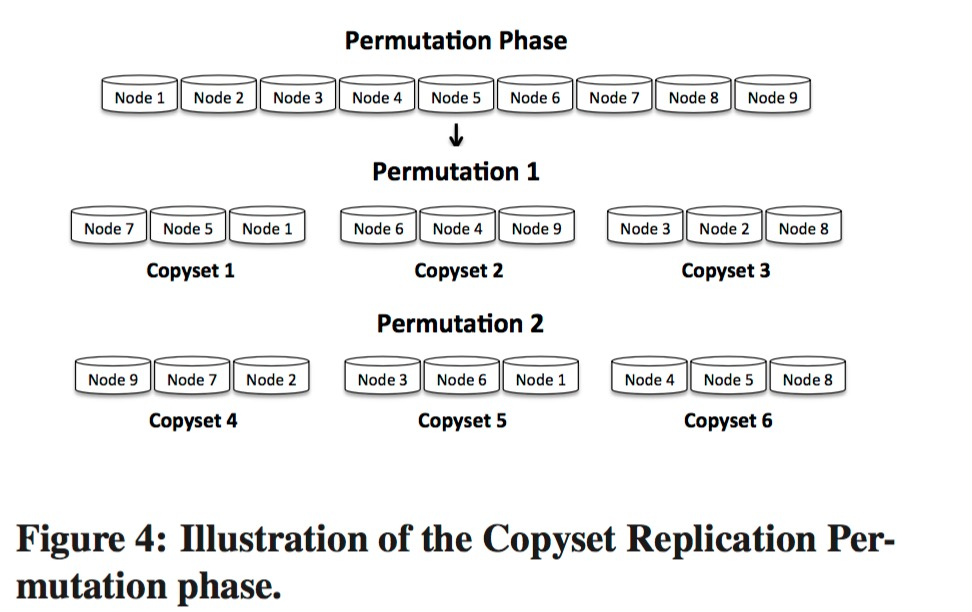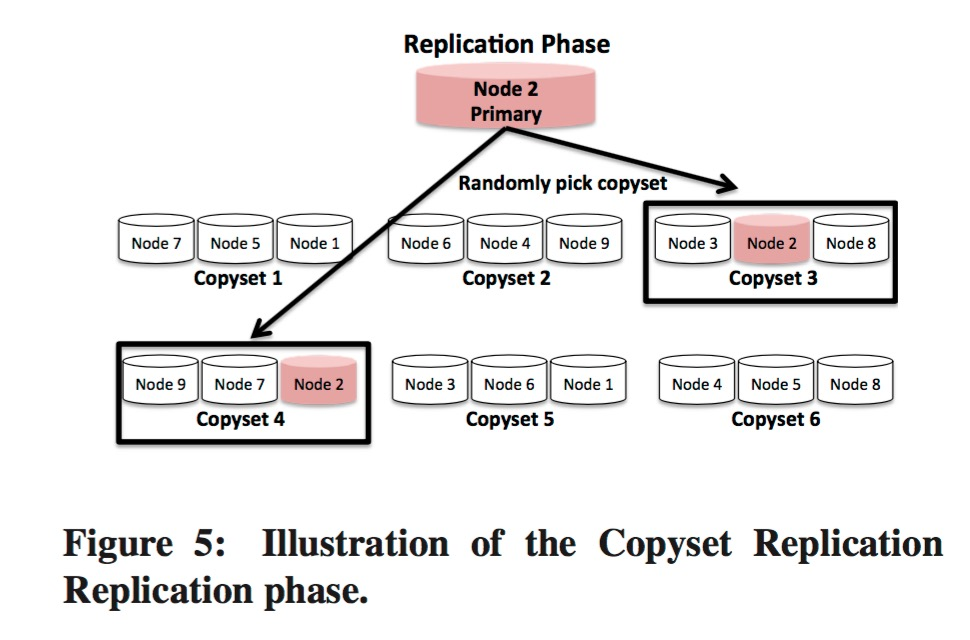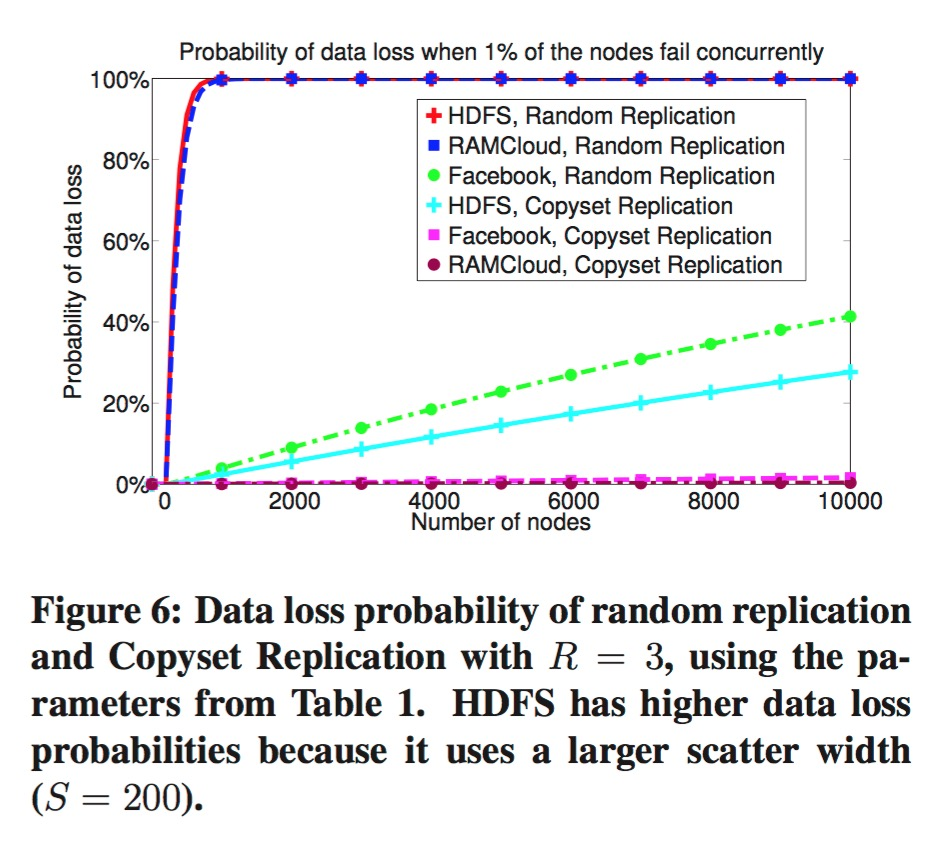# Copyset与多副本

2018/08/07• 如果副本分布的$$S$$太小，则故障恢复速度会慢，比如$$S$$为2，那个故障恢复时，只能从2个节点上恢复数据。
• 如果$$S$$太大，则会增加数据丢失的风险。因为当任意节点故障时，其波及的分片数随$$S$$的增大而增多。

• $$S$$ scatter width
• $$R$$ 副本数
• $$N$$ 集群实例数
• $$P$$ 副本排列组合次数

1. 排列阶段(Permutation phase)
2. 选择副本阶段(Replication phase)• 选定$$S$$和$$R$$
• 将集群实例排列$$P = \frac{S}{R-1}$$次，如果$$P$$不是一个整数，我们向上取整。
• 每次排列时，将集群节点完全随机排列，然后分为$$R$$组，每组为一个copyset。在排列阶段，也可以加入一些约束条件，比如不要让相同机架的节点比邻等
• 选取任意集群节点为第一个/主副本，然后随机选取一个包含该节点的copyset给对应的分片使用（上图中Replication phaseNode 2的箭头指向的copyset3copyset4的含义为这2个copyset都满足条件，随机选取一个即可）

[1, 6, 5, 3, 4, 8, 9, 7, 2]


[1, 6, 5], [3, 4, 8], [9, 7, 2]


[1, 6, 5], [3, 4, 8], [9, 7, 2]
[1, 2, 7], [5, 4, 6], [3, 9, 8]